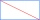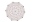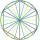# Diagonal + quadratic equation - math problems

#### Number of problems found: 26

• Perimeter and diagonalThe perimeter of the rectangle is 82 m, the length of its diagonal is 29 m. Find the dimensions of the rectangle.
• Block or cuboidThe wall diagonals of the block have sizes of √29cm, √34cm, √13cm. Calculate the surface and volume of the block.
• A rectangle 2A rectangle has a diagonal length of 74cm. Its side lengths are in ratio 5:3. Find its side lengths.
• Faces diagonalsIf a cuboid's diagonals are x, y, and z (wall diagonals or three faces), then find the cuboid volume. Solve for x=1.3, y=1, z=1.2
• Ratio of sidesCalculate the area of a circle with the same circumference as the circumference of the rectangle inscribed with a circle with a radius of r 9 cm so that its sides are in ratio 2 to 7.
• RectangleThere is a rectangle with a length of 12 cm and a diagonal 8 cm longer than the width. Calculate the area of a rectangle.
• Diamond diagonalsFind the diamond diagonal's lengths if the area is 156 cm2 and side is 13 cm long.
• Diagonals of a rhombus 2One diagonal of a rhombus is greater than the other by 4 cm. If the area of the rhombus is 96 cm2, find the side of the rhombus.
• DiagonalsWhat x-gon has 54 diagonals?
• Solid cuboidA solid cuboid has a volume of 40 cm3. The cuboid has a total surface area of 100 cm squared. One edge of the cuboid has a length of 2 cm. Find the length of a diagonal of the cuboid. Give your answer correct to 3 sig. Fig.
• Diamond diagonalsCalculate the diamonds' diagonals lengths if the diamond area is 156 cm square, and the side length is 13 cm.
• Surface area of the topA cylinder is three times as high as it is wide. The length of the cylinder’s diagonal is 20 cm. Find the surface area of the top of the cylinder.
• Diagonal 20Diagonal pathway for the rectangular town plaza whose length is 20 m longer than the width. if the pathway is 20 m shorter than twice the width. How long should the pathway be?
• Diamond diagonalsCalculate the diamond's diagonal lengths if its content is 156 cm2 and the side length is 13 cm.
• RectangleThe rectangle has a perimeter 75 cm. Diagonal length is 32.5 cm. Determine the length of the sides.
• Body diagonalThe cuboid has a volume of 32 cm3. Its side surface area is double as one of the square bases. What is the length of the body diagonal?
• Rectangle diagonalsIt is given a rectangle with an area of 24 cm2 a circumference of 20 cm. The length of one side is 2 cm larger than the length of the second side. Calculate the length of the diagonal. Length and width are yet expressed in natural numbers.
• Diagonal of the rectangleCalculate the rectangle's diagonal, which area is 54 centimeters square, and the circuit is equal to 30 cm.
• Polygon 42Which polygon has 42 more diagonals than sides?
• Sphere and coneWithin the sphere of radius G = 33 cm inscribe the cone with the largest volume. What is that volume, and what are the dimensions of the cone?

Do you have an exciting math question or word problem that you can't solve? Ask a question or post a math problem, and we can try to solve it.

We will send a solution to your e-mail address. Solved examples are also published here. Please enter the e-mail correctly and check whether you don't have a full mailbox.

Looking for help with calculating roots of a quadratic equation? Diagonal - math problems. Quadratic Equations Problems.